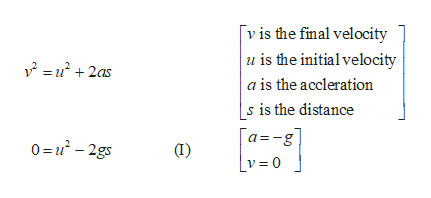# What is the maximun height attained by an object thrown straight up with an initial velocity of 80 m/s

Question
3 views

What is the maximun height attained by an object thrown straight up with an initial velocity of 80 m/s

check_circle

Step 1

The initial velocity is 80 m/s and at maximum height the velocity will be zero.

Write the kinematic expression for final velocity.help_outlineImage Transcriptionclosevis the final velocity u is the initialvelocity u2as + a is the accleration s is the distance a-g |v=0 0=-2gs fullscreen
Step 2

Rearrange equation (I) to obtain an expression fo...

### Want to see the full answer?

See Solution

#### Want to see this answer and more?

Solutions are written by subject experts who are available 24/7. Questions are typically answered within 1 hour.*

See Solution
*Response times may vary by subject and question.
Tagged in

### Physics